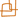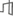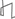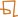IntroductionArchitecture, Systems & NetworksLanguage, Learning, Vision & GraphicsPhysical, Biological & Social SystemsTheory### Numerical Methods for Biomolecule Electrostatics

#### Jaydeep P. Bardhan, Michael D. Altman, Jacob K. White & Bruce Tidor

##### Introduction

Electrostatic interactions play important roles in biomolecule structure and function. In contrast to hydrogen bonding and van der Waals interactions, however, electrostatic interactions act over much longer distances. This long-range nature significantly complicates computational modeling.

Since computational expense prohibits explicitly modeling the many solvent molecules surrounding a biomolecule of interest, approximate models have been introduced that capture solvent effects in an average way . In these models, macroscopic laws of electrostatics are assumed to hold in the molecular interior and in the solvent region exterior; the charge distribution in the molecule is assumed to be a set of discrete point charges located at the atom centers, and the potential satisfies the Poisson equation in this region. In the solvent exterior, mobile water molecules and salt ions screen charges and the Poisson--Boltzmann equation governs the electrostatic potential.

Even these simplified models, however, require large quantities of computer time and memory. In this project we focus on designing new, more efficient numerical techniques for computing the electrostatic interactions between biomolecules and surrounding solvent.

##### Previous Work

Finite difference methods (FDM) are one of the most popular approaches for molecular electrostatics ; in these methods, a grid is laid down over the problem domain, and one finds a set of electrostatic potentials at the grid points that approximately solve the underlying partial differential equations. The finite difference approach is straightforward to understand and implement, but suffers from several sources of error. First, the irregular molecule-solvent interface cannot be accurately resolved on a regular grid. Second, the point charges that represent the molecular charge distribution must be spread to nearby grid points. Finally, the mathematical model has an infinite domain, which the finite difference method is forced to truncate.

The boundary element method (BEM) is another technique that can be used to model molecular electrostatics . The partial differential equations governing the potential throughout space are converted to an integral equation, or set of integral equations, over the molecular surface. The transformation therefore reduces the dimensionality of unknowns from three to two. In addition, the integral equation suffers from none of the grid-based inaccuracies inherent to finite difference methods: boundary conditions at infinity are treated exactly, point charges can be treated exactly, and the molecular surface can be represented much more accurately.

##### Approach

The linear systems that arise in the BEM are dense. Solving these systems by Gaussian elimination therefore has memory requirements that grow quadratically in time with respect to the number of unknowns, and time requirements grow cubically. For large systems, such cost is prohibitive, and we use Krylov subspace iterative methods such as GMRES instead. Using Krylov methods allows us to avoid the cubic time cost, but the dense matrix-vector multiply still limits performance. We therefore use techniques that compute the matrix-vector product approximately but in much less time and memory.

We have shown, for instance, that the precorrected-FFT algorithm  can be used to solve the BEM formulation of the biomolecule electrostatics problem . To reduce memory requirements, we have developed a new algorithm, which we call FFTSVD, for biomolecule electrostatics and bioMEMS simulations . The algorithm is based on compressing long-range interactions using an approximate singular value decomposition (SVD), and then quickly computing the interactions using the FFT.

##### Summary

The boundary element method holds significant promise for improving the accuracy and speed of biomolecule electrostatics simulations. We have already demonstrated the viability of a fast solver approach . We believe that the FFTSVD algorithm and future techniques will allow us to dramatically reduce the memory and time requirements for these calculations.

##### References

 M. K. Gilson, K. A. Sharp, and B. H. Honig. Calculating the electrostatic potential of molecules in solution: Method and error assessment. Journal of Computational Chemistry, 9:327-335, 1987.

 S. S. Kuo, M. D. Altman, J. P. Bardhan, B. Tidor, and J. K. White. Fast Methods for Biomolecule Electrostatics. International Conference on Computer-Aided Design (ICCAD), 2002.

 J. Phillips and J. K. White. A Precorrected-FFT Method for Electrostatic Analysis of Complicated 3-D Structures. IEEE Transactions on Computer-Aided Design of Integrated Circuits and Systems, 16:1059-1072, 1997.

 M. D. Altman, J. P. Bardhan, B. Tidor, and J. K. White. FFTSVD: A Fast Multi-Scale BEM Algorithm Suitable for BioMEMS Design. submitted to IEEE Transactions on Computer-Aided Design of Integrated Circuits and Systems.Computer Science and Artificial Intelligence Laboratory (CSAIL) The Stata Center, Building 32 - 32 Vassar Street - Cambridge, MA 02139 - USA tel:+1-617-253-0073 - publications@csail.mit.edu (Note: On July 1, 2003, the AI Lab and LCS merged to form CSAIL.)